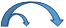# Distributive Property

The distributive Property states that you can distribute multiplication over addition and subtraction.

In English to distribute means to dispense or dole out.

In math, the distributive property allows us to multiply before performing operations within the parenthesis. We also use distributive property to multiply numbers mentally.

For example: 2(10 + 3) means 2 × (10 + 3)

2 is distributed to 10 and to 32(10 + 3) = 2 × 10 + 2 × 3

= 20 + 6

= 26

Example: Use the distributive property to evaluate 3(6 + 5).3(6 + 5) = 3 × 6 + 3 × 5

= 18 + 15

=33

Example: Use the distributive property to evaluate 8(2 + 5).8(2 + 5) = 8 × 2+ 8 × 5

= 16 + 40

= 56

Example: Mental math. Note that 14 = 10 + 4. In order to find 6 × 14 mentally, use the distributive property to find 6(10 + 4).6(10 + 4) = 6 × 10 + 6 × 4

=60 + 24

= 84

Example: Use the distributive property to evaluate 5(4 - 1).5(4 - 1) = 5 × 4 - 5 × 1

= 20 - 5

= 15

Example: Mental math. Note that 18 = 20 - 2. In order to find 4 × 18 mentally, use the distributive property to evaluate 4(20 - 2).4(20 - 2) = 4 × 20 - 4 × 2

= 80 - 8

= 72

Example: Mental math. Note that 17 = 20 - 3. In order to find 7 × 17 mentally, use the distributive property to evaluate 7(20 - 3).7(20 - 3) = 7 × 20 - 7 × 3

=140 - 21

= 119

Now that you know how to use the distributive property you can manipulate numbers in order to do mental math.

Math
algebra
Inverse Properties of Addition and Multiplication
Trichotomy Property

To link to this Distributive Property page, copy the following code to your site: